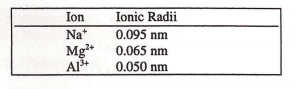# Problem: The radii of the ions in this series decrease because a) the elements are in the same period. b) the effective nuclear charge is increasing. c) the atomic radius of Na decreases  from Na to Al. d) the first ionization energies increase from Na to Al.

###### FREE Expert Solution
79% (382 ratings)
###### Problem Details

The radii of the ions in this series decrease because

a) the elements are in the same period.

b) the effective nuclear charge is increasing.

c) the atomic radius of Na decreases  from Na to Al.

d) the first ionization energies increase from Na to Al.What scientific concept do you need to know in order to solve this problem?

Our tutors have indicated that to solve this problem you will need to apply the Periodic Trend: Effective Nuclear Charge concept. You can view video lessons to learn Periodic Trend: Effective Nuclear Charge. Or if you need more Periodic Trend: Effective Nuclear Charge practice, you can also practice Periodic Trend: Effective Nuclear Charge practice problems.

What is the difficulty of this problem?

Our tutors rated the difficulty ofThe radii of the ions in this series decrease because a) th...as medium difficulty.

How long does this problem take to solve?

Our expert Chemistry tutor, Jules took 2 minutes and 14 seconds to solve this problem. You can follow their steps in the video explanation above.

What professor is this problem relevant for?

Based on our data, we think this problem is relevant for Professor Chang's class at VCU.